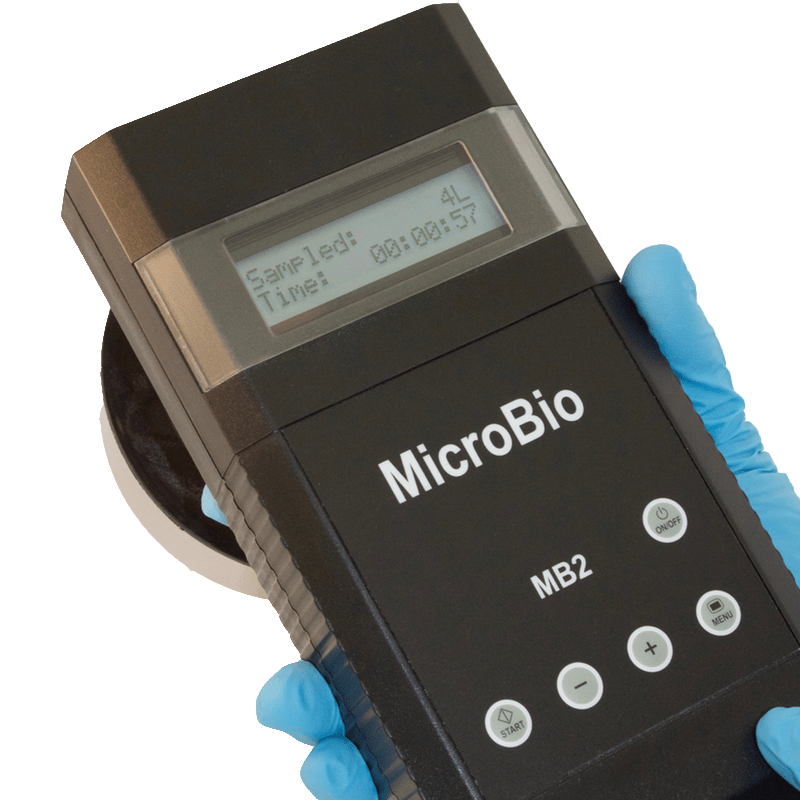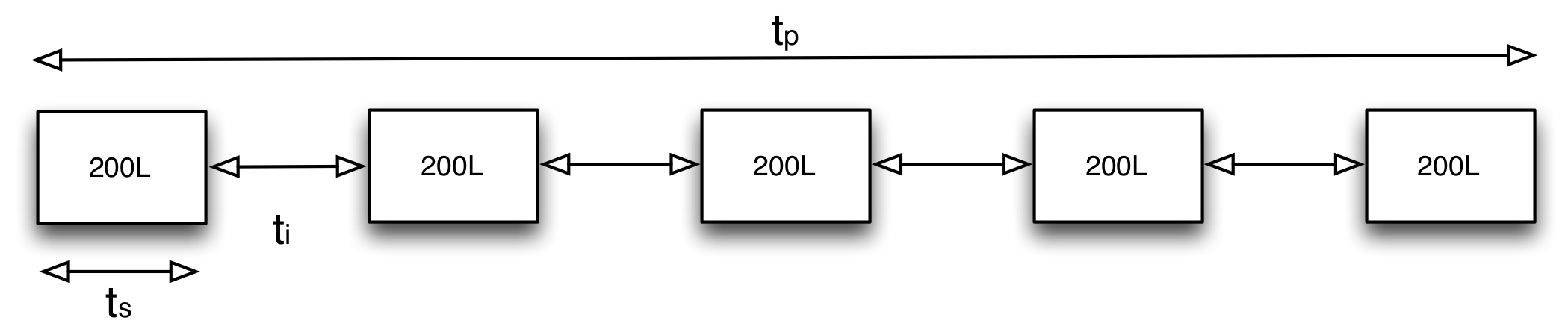# Sequential Sampling on MicroBio MB2 SamplersSequential sampling enables a programmable volume of air to be sampled over an extended period of time. This is useful in clean room environments where contamination levels are normally low, resulting in low counts. Monitoring for short periods could risk missing potential hazards that may occur during the day. The MicroBio MB2 can now be set up and left to work autonomously, sequential sampling up to 10,000 litres over a 24 hour period.

Capable of sampling at 100 litres per minute, while maintaining impaction velocities within the upper limit of 20 m/s for maximum viability of organisms, the MicroBio MB2 will help users achieve ISO 14695 air sampling requirements.

## Sequential Sampling – How it works

A cubic meter of air would normally be sampled in 10 minutes. Sequential sampling allows the user to configure the total volume to be sampled, by using an adjustable volume or one of the user preset volumes, over 5 minutes to 24 hours with optional delayed start if required.

To illustrate how sequential sampling works, consider a 1,000 litre sample taken over an 8 hour period (tp), broken into five separate samples (n). These numbers are entered into the MicroBio MB2 following a simple set of questions. The MicroBio MB2 then calculates how much air needs sampling per sequence (ts being the time to take each sample) and the time between the samples (ti), calculated using equation 1:Equation 1: Interval time calculation

The sampling sequence illustrated in figure 1, shows how the sample is broken down into five smaller samples of 200 litres each.  ts for a standard MicroBio MB2 will be 2 minutes for each 200 litres of air.Figure 1: Example sequence

Using equation 1, the interval between each of these 200 litre samples is calculated as 7,050 seconds or 1 hour, 57 minutes and 30 seconds. At the end of the sampling sequence the MicroBio MB2 displays a finished message then automatically powers off. When the MicroBio MB2 is next switched on, it will display a finished message with the total volume of air sampled.